# SAT Math Multiple Choice Question 191: Answer and Explanation

### Test Information

Question: 191

11.=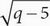The equation above can be solved for two solutions, one of which is extraneous. What is the value of the extraneous solution?

• A. 5
• B. 6
• C. 8
• D. 9

B An extraneous solution is an answer that when plugged back into the equation causes the equation to be false. Begin by factoring and reducing the fraction on the left side of the equation to get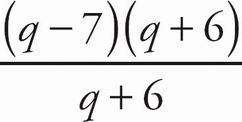=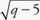or q - 7 =. Square both sides of the equation to get q2 - 14q + 49 = q - 5. Set the equation to 0 to get q2 - 15q + 54 = 0. Factor the quadratic to get (q - 9)(q - 6) = 0. Therefore, q = 9 or q = 6. Eliminate (A) and (C) because neither ofthese answers is a possible solution for q. Plug 6 in for q in the equation to see if this value of q works. The equation becomes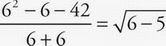. Solve both sides of the equation to get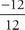= 1. Since this statement is not true, 6 is the extraneous solution. The correct answer is (B).